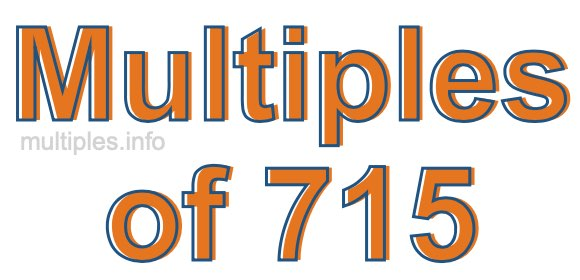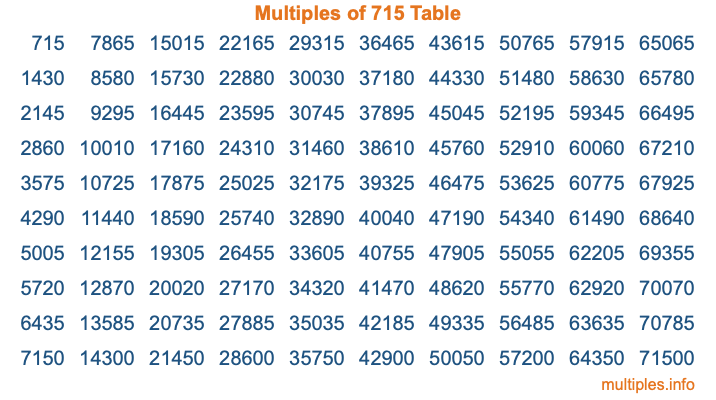Multiples of 715Welcome to the Multiples of 715 page. Here we will first teach you everything you will ever need to know about the multiples of 715, and then give you a study guide summary of everything we taught you to make sure you remember it all. Use this page to look up facts and learn information about the multiples of 715. This page will make you a multiples of seven hundred fifteen expert!

Definition of Multiples of 715
Multiples of 715 are all the numbers that when divided by 715 equal an integer. Each of the multiples of 715 are called a multiple. A multiple of 715 is created by multiplying 715 by an integer.

Therefore, to create a list of multiples of 715, you start with 1 multiplied by 715, then 2 multiplied by 715, then 3 multiplied by 715, and so on for as long as you want. Thus, the list of the first five multiples of 715 is 715, 1430, 2145, 2860, and 3575. To see a larger list of multiples of 715, see the printable image of Multiples of 715 further down on this page. We also have a category where you can choose any nth multiple of 715.

Multiples of 715 Checker
The Multiples of 715 Checker below checks to see if any number of your choice is a multiple of 715. In other words, it checks to see if there is any number (integer) that when multiplied by 715 will equal your number. To do that, we divide your number by 715. If the the quotient is an integer, then your number is a multiple of 715.

Is  a multiple of 715?

Least Common Multiple of 715 and ...
A Least Common Multiple (LCM) is the lowest multiple that two or more numbers have in common. This is also called the smallest common multiple or lowest common multiple and is useful to know when you are adding our subtracting fractions. Enter one or more numbers below (715 is already entered) to find the LCM.

Check out our LCM Calculator if you need more details about the Least Common Multiple or if you need the LCM for different numbers for adding and subtraction fractions.

nth Multiple of 715
As we stated above, 715 is the first multiple of 715, 1430 is the second multiple of 715, 2145 is the third multiple of 715, and so on. Enter a number below to find the nth multiple of 715.

th multiple of 715

Multiples of 715 vs Factors of 715
715 is a multiple of 715 and a factor of 715, but that is where the similarities end. All postive multiples of 715 are 715 or greater than 715. All positive factors of 715 are 715 or less than 715.

Below is the beginning list of multiples of 715 and the factors of 715 so you can compare:

Multiples of 715: 715, 1430, 2145, 2860, 3575, etc.

Factors of 715: 1, 5, 11, 13, 55, 65, 143, 715

As you can see, the multiples of 715 are all the numbers that you can divide by 715 to get a whole number. The factors of 715, on the other hand, are all the whole numbers that you can multiply by another whole number to get 715.

It's also interesting to note that if a number (x) is a factor of 715, then 715 will also be a multiple of that number (x).

Multiples of 715 vs Divisors of 715
The divisors of 715 are all the integers that 715 can be divided by evenly. Below is a list of the divisors of 715.

Divisors of 715: 1, 5, 11, 13, 55, 65, 143, 715

The interesting thing to note here is that if you take any multiple of 715 and divide it by a divisor of 715, you will see that the quotient is an integer.

Multiples of 715 Table
Below is an image of the first 100 multiples of 715 in a table. The table is in chronological order, column by column. The first column has the first ten multiples of 715, the second column has the next ten multiples of 715, and so on.The Multiples of 715 Table is also referred to as the 715 Times Table or Times Table of 715. You are welcome to print out our table for your studies.

Negative Multiples of 715
Although not often discussed or needed in math, it is worth mentioning that you can make a list of negative multiples of 715 by multiplying 715 by -1, then by -2, then by -3, and so on, to get the following list of negative multiples of 715:

-715, -1430, -2145, -2860, -3575, etc.

Multiples of 715 Summary
Below is a summary of important Multiples of 715 facts that we have discussed on this page. To retain the knowledge on this page, we recommend that you read through the summary and explain to yourself or a study partner why they hold true.

There are an infinite number of multiples of 715.

A multiple of 715 divided by 715 will equal a whole number.

715 divided by a factor of 715 equals a divisor of 715.

The nth multiple of 715 is n times 715.

The largest factor of 715 is equal to the first positive multiple of 715.

715 is a multiple of every factor of 715.

715 is a multiple of 715.

A multiple of 715 divided by a divisor of 715 equals an integer.

715 divided by a divisor of 715 equals a factor of 715.

Any integer times 715 will equal a multiple of 715.

Multiples of a Number
Here you can get the multiples of another number, all with the same attention to detail as we did for multiples of 715 on this page.

Multiples of
Multiples of 716
Did you find our page about multiples of seven hundred fifteen educational? Do you want more knowledge? Check out the multiples of the next number on our list!